#### You may also like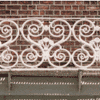### Frieze Patterns in Cast Iron

A gallery of beautiful photos of cast ironwork friezes in Australia with a mathematical discussion of the classification of frieze patterns.### The Frieze Tree

Patterns that repeat in a line are strangely interesting. How many types are there and how do you tell one type from another?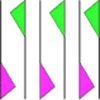### Friezes

Some local pupils lost a geometric opportunity recently as they surveyed the cars in the car park. Did you know that car tyres, and the wheels that they on, are a rich source of geometry?

# ...on the Wall

##### Age 11 to 14Challenge Level

Isabel from St Andrew's Scots School in Argentina used Geogebra to investigate the problem. This is Isabel's work, with some teacher comments.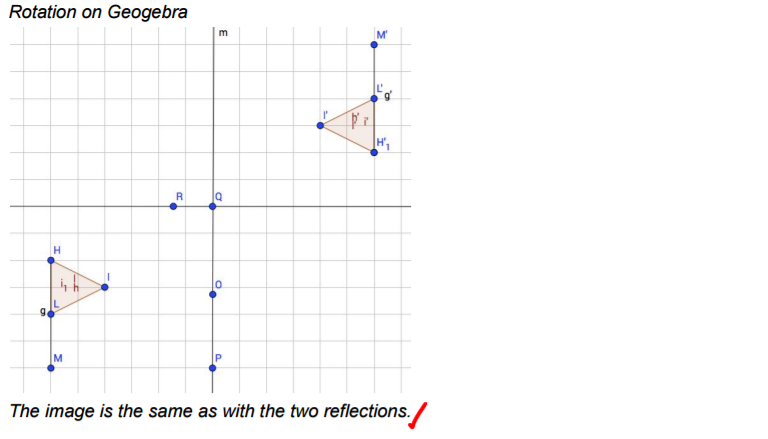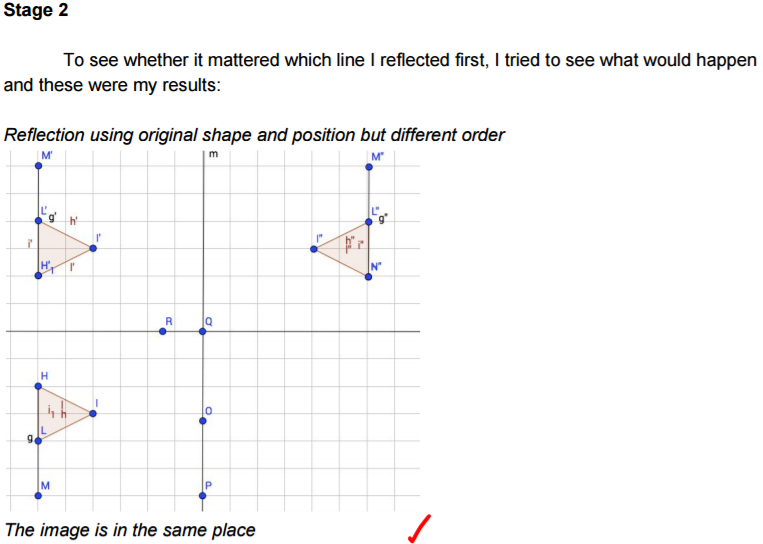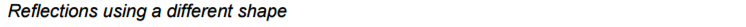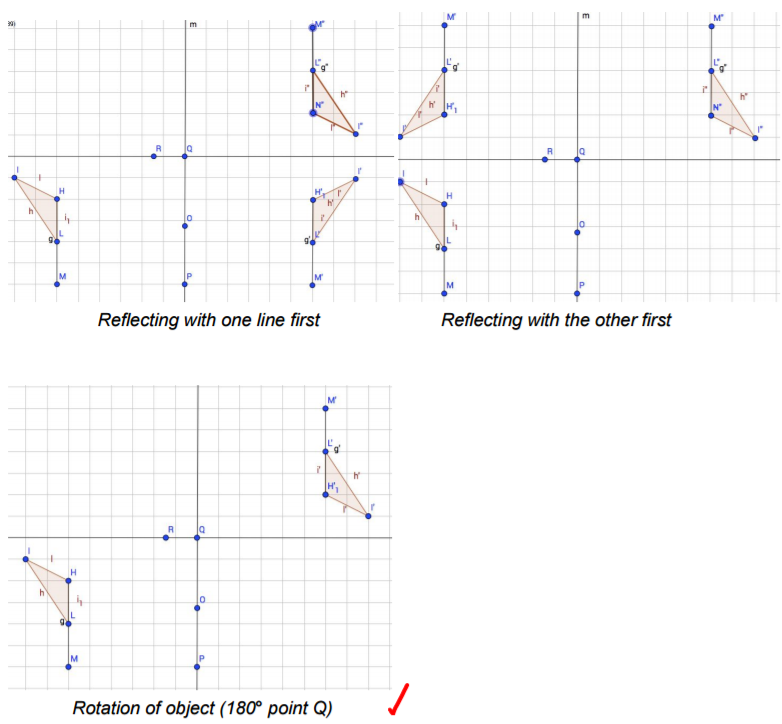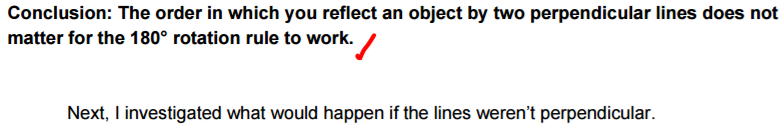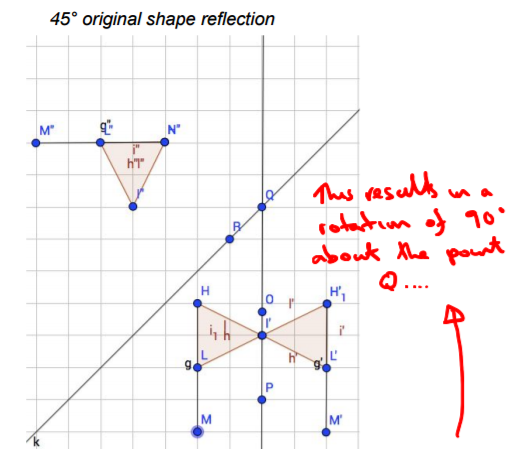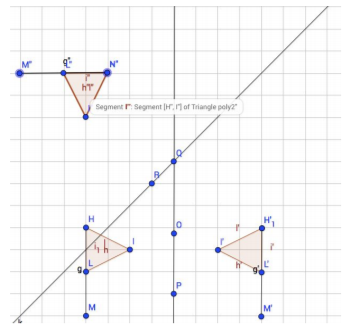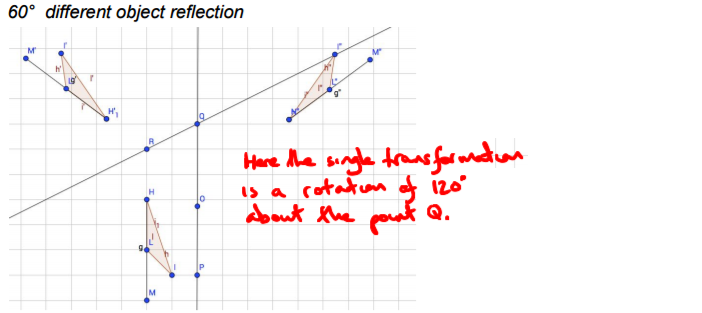Wenchi, also from St Andrew's Scots School, noticed a pattern for mirror lines that are not perpendicular:
There is a pattern when the mirror lines cross different angles. Reflecting in 2 is equal to 1 rotation by $2\theta$ about the point of intersection of the lines, where $\theta$ is the angle between the lines.
When the lines cross at $45^\text o$, the object rotates by $90^\text o$, and when the lines cross at $60^\text o$, the object rotates $120^\text o$.

Notice that applying this rule to lines which cross at $90^\text o$ gives a rotation of $180^\text o$, as we have already seen.

Here is our explanation of the transformations required in the general case of mirror lines at any angle :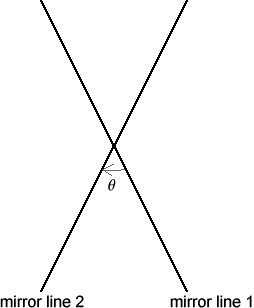The resulting transformation is a rotation by $2\theta$ about the point of intersection of the lines. If the direction of the angle $\theta$ is from the first line of reflection to the second, then the direction of rotation is the same as the direction of $\theta$.

You should probably check that the same thing works if you reflect in the further line first (remembering that this means the direction is reversed), and if the flag is between the two lines.

We just need to check the result for a single point, because then every point will rotate by the same amount and so the flag will remain intact (and will have rotated by the same amount).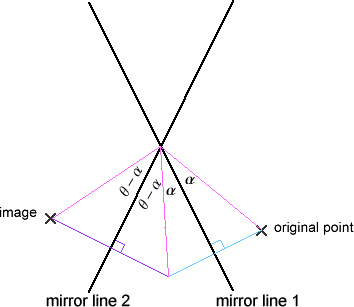The diagram says it all, really: the line segment joining the point to the origin has rotated by an angle of $\alpha+\alpha+(\theta-\alpha)+(\theta -\alpha)=2\theta$ in the direction of $\theta$.